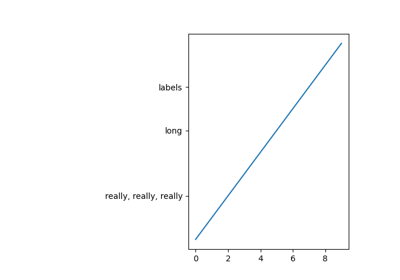# matplotlib.figure.SubplotParams¶

class matplotlib.figure.SubplotParams(left=None, bottom=None, right=None, top=None, wspace=None, hspace=None)[source]

Bases: object

A class to hold the parameters for a subplot.

All dimensions are fractions of the figure width or height. Defaults are given by rcParams["figure.subplot.[name]"].

Parameters: leftfloatThe left side of the subplots of the figure. rightfloatThe right side of the subplots of the figure. bottomfloatThe bottom of the subplots of the figure. topfloatThe top of the subplots of the figure. wspacefloatThe amount of width reserved for space between subplots, expressed as a fraction of the average axis width. hspacefloatThe amount of height reserved for space between subplots, expressed as a fraction of the average axis height.
update(self, left=None, bottom=None, right=None, top=None, wspace=None, hspace=None)[source]

Update the dimensions of the passed parameters. None means unchanged.

## Examples using matplotlib.figure.SubplotParams¶